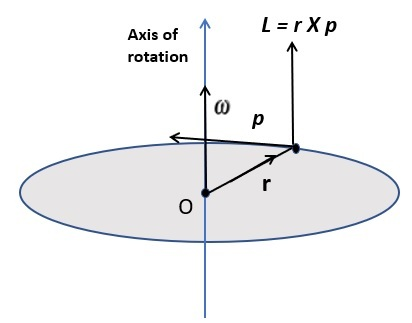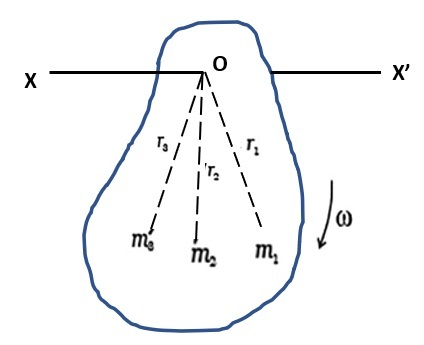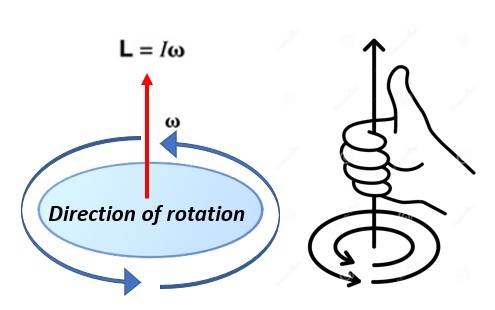# Angular Momentum- Definition, Formula, Unit, FAQs

## Introduction: What is Momentum?

Angular Momentum and Linear Momentum are vector quantities. The physical quantities which contain both magnitude and direction are called vector quantities. Ex: Displacement, Velocity, momentum, etc. Momentum is the product of the velocity and mass of the particle. In linear motion, it was denoted as linear momentum and it is expressed as P.

$$\mathrm{Linear\:momentum = mass\:\times\: velocity}$$

## Angular Momentum

If a particle moves in a circular path, then it is said to be in circular motion. The momentum of a particle in a circular motion is called angular momentum. The total Angular momentum of the particle is constant for a closed path. Angular momentum is defined as the product of the radius of the circular path and linear momentum. Its unit is $\mathrm{Kg\:m^2 s^{−1}}$. Dimensional formula of angular momentum is $\mathrm{[M\:L^2\:T^{−1}]}$.

$$\mathrm{\overrightarrow{L} =\overrightarrow{r}\:\times\: \overrightarrow{P}}$$

or

$$\mathrm{\overrightarrow{L}= \overrightarrow{I}\:\times\:\overrightarrow{\omega}}$$Here,

p - linear momentum

I - a moment of inertia

ω - angular velocity

Velocity of the particle is the rate of change of distance (S). Similarly, angular velocity is the angular displacement (θ) of the particle per second(t). Linear velocity and angular velocity are related by

$$\mathrm{v=rω}$$

## Angular Momentum Formula

Let us consider a rigid body having n number particles rotating about an axis perpendicular to it. Masses of each particle are $\mathrm{m_1 ,m_2,m_3...................m_n}$ and their distances from the axis of rotation are $\mathrm{\overrightarrow{r_1},\overrightarrow{r_2},\overrightarrow{r_3}.................\overrightarrow{r_n}}$. As the particle position does not change, they rotate with the same angular velocity ω.

The velocity of the particle 1 in rotational motion is $\mathrm{v_1=r_1\:ω}$

Similarly for particles 2,3,4......................................n are

$\mathrm{v_2 = r_2 ω,\:v_3 = r_3 ω , …………. v_n = r_n\:ω}$.Linear momentum of the particle 1 is $\mathrm{p_1 = m_1 v_1}$

Similarly linear momentum of particles 2,3........................n are $\mathrm{p_2 = m_2 v_2,\:p_3 = m_3 v_3,\:and\:so\:on\:p_n = m_n v_n.}$

The angular momentum of the first particle is $\mathrm{L_1=r_1\:p_1}$

Similarly angular momentum of particles 2,3..............n are $\mathrm{L_2 = r_2 p_2, \:L_3 = r_3 p_3, ……… L_n = r_n p_n}$.

Substituting the value for $\mathrm{p_1,p_2,p_3..........................p_n}$.

$$\mathrm{L_1=r_1 m_1 v_1}$$

$$\mathrm{L_2=r_2 m_2 v_2}$$

$$\mathrm{L_3=r_3 m_3 v_3}$$

$$\mathrm{L_n=r_n m_n v_n}$$

Total angular momenta of the body is

$$\mathrm{L=r_1 m_1 v_1+r_2 m_2 v_2+r_3 m_3 v_3+...................r_n m_n v_n}$$

Now substituting the value for $\mathrm{v_1,v_2,v_3.......................v_n}$

$$\mathrm{L=m_1 r_1^2 \omega+m_2 r_2^2 \omega+m_3 r_3^2 \omega+.................+m_n r_n^2 \omega}$$

$$\mathrm{L=(m_1 r_1^2+m_2 r_2^2+m_3 r_3^2+................................m_n r_n^2)\omega}$$

$$\mathrm{L = I \omega}$$

$$\mathrm{I=m_1 r_1^2+m_2 r_2^2+m_3 r_3^2+...........................+m_n r_m^2}$$

This is required equation for moment of inertia of the body about its axis of rotation.

## Right-Hand Thumb Rule

Right-hand rule gives the direction of angular momentum. If the four fingers except the thumb finger are folded and show the direction of rotation of the body then the thumb finger pointed upward shows the direction of angular momentum, angular velocity, and torque.## Angular Momentum and Torque

Angular momentum is defined as the moment of linear momentum. Torque is the force that tends the object to rotate about an axis. Torque is also a vector quantity. Unit of Torque in Nm.

$$\mathrm{\overrightarrow{\tau}=\overrightarrow{r}\times \overrightarrow{F}=rFsin\theta}$$

For rotating body,

$$\mathrm{\overrightarrow{\tau}=\overrightarrow{I}\times \overrightarrow{\alpha}}$$

Here

$\mathrm{\overrightarrow{\tau}}$= torque

$\mathrm{\overrightarrow{I}}$=moment of inertiaI

$\mathrm{\overrightarrow{\alpha}}$=angular acceleration

## Torque of a Rigid Body

Let us assume a rigid body containing n number of particles rotating about an axis passing through it with- angular momentum ω. Masses of each particle are $\mathrm{m_1, m_2,....... m_n}$ and their distances from the axis of rotation are $\mathrm{r_1,r_2..................r_n}$. As it is a rigid body angular momentum of the particles are the same as ω but linear velocity of the particles are different as $\mathrm{v_1,v_2..........................v_n}$.

Angular acceleration of the particle 1 is

$$\mathrm{a_1=\frac{dv_1}{dt}=\frac{d(r_1 ω)}{dt}\:\:\:\:\:(\frac{dr}{dt}=v=rω)}$$

$$\mathrm{a_1=r_1\frac{dω_1}{dt}= r_1\:\alpha\:\:\:\:\:(\frac{dω}{dt}=\alpha)}$$

Similarly for particle 2,.... n are

$$\mathrm{a_2 = r_2\:\alpha}$$

$$\mathrm{a_n = r_n\:\alpha}$$

Force acting on the particle 1 is

$$\mathrm{\overrightarrow{F_1}=m_1\overrightarrow{a_1}=m_1\overrightarrow{r_1}\alpha}$$

Forces on particles 2,..... n are

$$\mathrm{\overrightarrow{F_2}=m_2\overrightarrow{r_2}\overrightarrow{\alpha}..............\overrightarrow{F_n}=m_n\overrightarrow{r_n}\overrightarrow{\alpha}}$$

Torque of the particle 1 is

$$\mathrm{\overrightarrow{\tau_1}=\overrightarrow{r_1}\times \overrightarrow{F_1}=\overrightarrow{r_1}\times m_1\overrightarrow{r_1}\overrightarrow{\alpha}}$$

$$\mathrm{\overrightarrow{\tau_1}=m_1r_1^2\alpha}$$

Similarly for particles 2,.....n are

$$\mathrm{\overrightarrow{\tau_1}=m_2 r_2^2 \alpha}$$

$$\mathrm{\overrightarrow{\tau_n}=m_n r_n^2 \alpha}$$

Total torque of the rigid body is

$$\mathrm{\overrightarrow{\tau}=m_1 r_1^2 \alpha+ m_2 r_2^2 \alpha+.......................+m_n r_n^2 \alpha. }$$

$$\mathrm{\overrightarrow{\tau}=(m_1 r_1^2+m_2 r_2^2+.......................+m_n r_n^2)\alpha}$$

$$\mathrm{\overrightarrow{\tau}=I\alpha}$$

$$\mathrm{Torque = moment\:of\:inertia \times angular\:acceleration}$$

## Relation between Angular Momentum and Torque

Torque of rigid rotating body is,

$$\mathrm{\overrightarrow{\tau}=\overrightarrow{I}\times \overrightarrow{\alpha}}$$

$$\mathrm{\overrightarrow{\tau}=\overrightarrow{I}\times \frac{d\overrightarrow{\omega}}{dt}\:\:\:\:\:\:\:(\alpha = dω/dt )}$$

$$\mathrm{\overrightarrow{\tau}=\frac{d(\overrightarrow{I}\times \overrightarrow{\omega})}{dt}\:\:\:\:\:\:(\overrightarrow{L}=\overrightarrow{I}\times \overrightarrow{\omega})}$$

$$\mathrm{\overrightarrow{\tau}=\frac{d\overrightarrow{L}}{dt}}$$

Torque of a rotating body is equal to the change in angular momentum with respect to time. This is denoted as Newton’s second law on rotation.

## Examples for Angular Momentum

Angular motion is very much important in kinematics in physics. The applications of angular motion are abundant. Here are some examples which use angular momentum.

### Ice skater

In skating the player should maintain their angular velocity for their stability to make different patterns of the circular paths. To decrease the angular velocity of their paths they stretch their hands and to increase they come closer.

### Rotation of Earth

Rotation of the earth is the best example of angular momentum. Earth revolves around the sun due to orbital angular momentum. Earth rotates about its own axis due to spin angular momentum.

### Gyroscope

It is a device used to maintain the orientation and angular velocity based on the principle of conservation of angular momentum. The axis of rotation of the Gyro wheels remains in a fixed direction while an external force is applied to it. It is used in spaces where fixed orientation is needed. Angular momentum produced due to their fast-spinning makes the gyroscope stand straight on its axis.

## Conclusion

Angular momentum is a vector quantity that has both magnitude and direction. Angular momentum is calculated from the velocity and mass of the particle. Its unit is $\mathrm{Kg\:m^2\:s^{−1}}$. Right hand rule best explains the direction of angular momentum. Torque is also a vector quantity that makes the object rotate about an axis. Angular momentum has a wide range of applications in day-to-day life. The rotation of the earth is the best example of angular momentum.

## FAQs

Q1. What is the difference between momentum and angular momentum?

Ans.

Linear MomentumAngular momentum
Property of changing their position with respect to reference pointProperty of changing their angle with respect to the reference point.
Formulae:

$\mathrm{\overrightarrow{p}=m\times \overrightarrow{v}}$

Formulae:

$\mathrm{\overrightarrow{L}=\overrightarrow{I}\times \overrightarrow{\omega}}$

Force = mass x linear accelerationTorque = moment of inertia x angular acceleration
It is constant as long as constant force is applied.4. It is constant as long as constant torque is applied.
$\mathrm{Unit - Kg\:m^2\:s^{−1}}$$\mathrm{Unit - Kg\:m^2\:s^{−1}}$

Table-1: Difference between Linear momentum and Angular momentum

Q2. What is the Law of Conservation of angular momentum?

Ans. Angular momentum of the body is constant until then torque is applied to it. This is the law of conservation of angular momentum.

Q3. Divers change their position before and after diving. Why?

Ans. Before diving from the diving board the diver stretches their hands and legs fully to maintain a large moment of inertia. After diving, they drew their hands and legs in a closed position to reduce the moment of inertia. Thus, the driver spins fast due to the low moment of inertia. Again, while reaching the water, they stretched their body.

Q4. How does an ice-skater control their speed?

Ans. While spinning the ice- skater stretches their hand or makes their hand close. They did this to decrease or increase their spinning speed.

Q5. Explain torque with an example?

Ans. Torque is a rotational force. While opening and closing the water taps in our home, we are giving a torque that makes it rotate. Shorter handles are harder to open while langer handles are easy to do so.

Updated on: 18-Apr-2023

144 Views